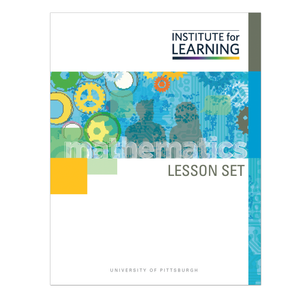# Solving Problems Using Linear and Exponential Models (Algebra 1)

Regular price \$6.25 \$0.00 Unit price per

In this lesson set, students will develop their understanding of exponential functions by building and analyzing exponential functions, introduced in both real-world and mathematical contexts, and by comparing them with linear functions. They will then apply their understanding of linear and exponential functions as they solve problems presented using a variety of representations.

Through engaging in this lesson set, students will:

• examine the way that exponential functions grow and compare this to the growth of linear functions;
• build linear and exponential function rules from contexts and tables of values for both increasing and decreasing functions;
• graph linear and exponential functions and connect features of these graphs to the parameters in the function rules; and
• determine whether situations can be modeled by linear or exponential functions and identify distinguishing features of these situations.

Standards: F-LE.A.1, F-LE.A.1.A, F-LE.A.1.B, F-LE.A.1.C, F-LE.A.2, F-LE.B.5